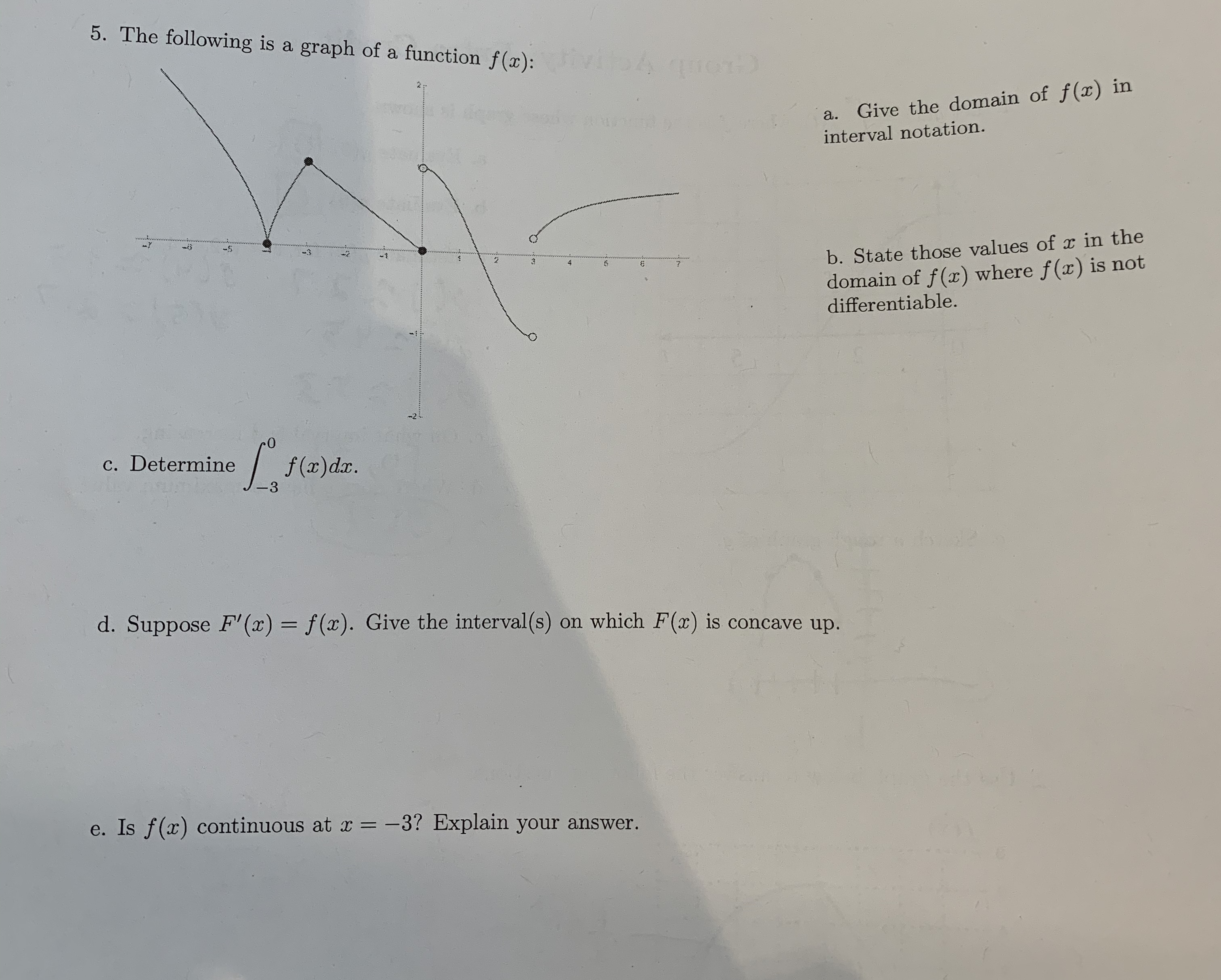# 5. The following is a graph of a function f(z): ivi DA cmcGive the domain of f(x) inunctsа.interval notation.b. State those values of r in thedomain of f(x) where f() is notdifferentiable.23-2c. Determinef(a)dar.3d. Suppose F' (x)f(x). Give the interval(s) on which F(x) is concave up.e. Is f(x) continuous at= -3? Explain your answer.

Question
39 viewshelp_outlineImage Transcriptionclose5. The following is a graph of a function f(z): ivi DA cmc Give the domain of f(x) in uncts а. interval notation. b. State those values of r in the domain of f(x) where f() is not differentiable. 2 3 -2 c. Determine f(a)dar. 3 d. Suppose F' (x) f(x). Give the interval(s) on which F(x) is concave up. e. Is f(x) continuous at = -3? Explain your answer. fullscreen
check_circle

Step 1

Hey, since there are multiple subparts questions posted, we will answer three subparts. If you want any specific question to be answered then please submit that question only or specify the question number in your message

Step 2

a) The domain of the given graph is –7<x<7.

Step 3

b) The function f(x) is not differentiable in the...

### Want to see the full answer?

See Solution

#### Want to see this answer and more?

Solutions are written by subject experts who are available 24/7. Questions are typically answered within 1 hour.*

See Solution
*Response times may vary by subject and question.
Tagged in

### Functions